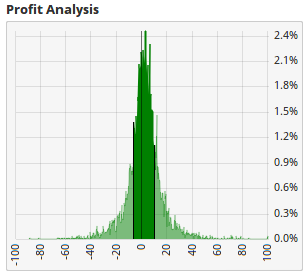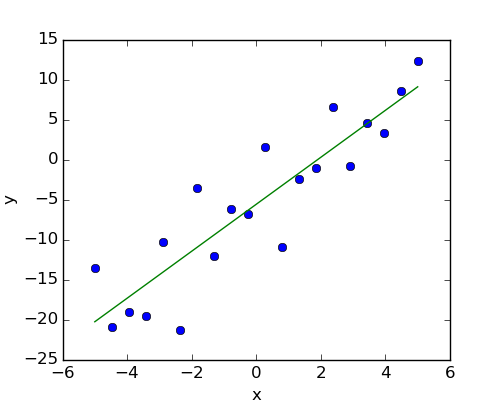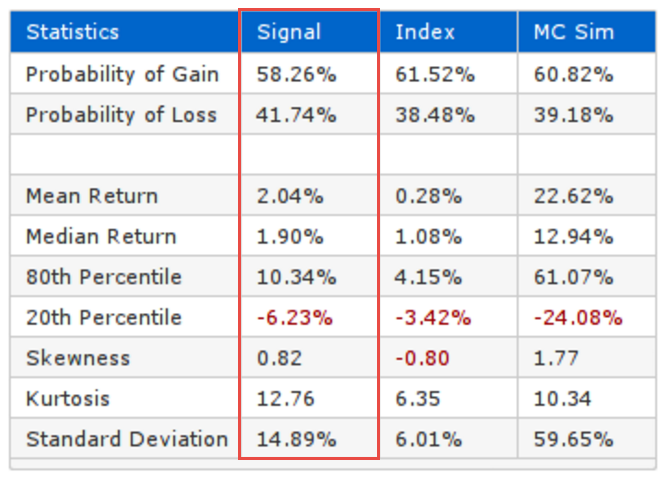Select Page

Death by a thousand small losses—the truth about statistics

Sep 9, 2016

Last time in Lies, Damned Lies, and Average Returns, I introduced the work we’ve been doing with the Optuma Signal Tester. I revealed how a simple average return (in tests or actual results) is an incomplete picture that can lead to a deceptive measure of performance. We need to look deeply into the average to get to the true results. Make sure you read it first if you haven’t already, as this post follows on.

Lingchi (凌迟; 凌遲) is an old Chinese execution translated as “Death by a Thousand Cuts”. I’ll continue with the explanation of how important disciplined testing is, and how critical a clear understanding of all the results are. When we don’t understand all the numbers that make up our test results, we may be forced to endure more small losses than we can survive. Or “Death by a thousand small losses”.

A Confession

First, I have a confession to make. My engineering degree was full of hard core math. We did three years of Calculus, Fourier, Taylors, Integrals, etc. I got through it all, but there was one subject I failed. I retook the final exam and even failed that. I’m sure you’ve guessed by now it was Statistics. In the end the engineering faculty gave me what they called a “faculty pass”. Maybe they thought it was not worth the effort sending me back! This left me with an incredible brain block that caused me to rock back and forth in the corner whenever anyone mentioned the “s” word. It’s with a significant amount of irony that much of the innovation I work on now is based on statistics. You see, once I started to apply statistics, it began to make sense. If statistics confuse you, or scare you—don’t worry—we’ll get to the most important things that you need to know.

Recap

Last time we introduced the Profit Analysis plot as part of the Optuma Signal Tester. Every time you are quoted an average, you need to remember that behind that number is a plot like this. It helps us to see what the probability of repeating that average return in the future is.

In this post I cover all the statistics that we list in the Signal Tester, and explain why they are important.Where’s that Soapbox?

First, I need to jump onto the soapbox for a minute to address that dreaded statement we hear in finance. You know the one: “Past Returns are not an indicator of Future Performance”. Rubbish, Trash or Bollocks! (whichever word makes sense to you).

If you’re investing in a person or Portfolio Manager who got “lucky”—then yes—that’s a very true statement. But what we do with Technical Analysis is very different. The whole premise of Technical Analysis is probability and an expectation that the past will repeat into the future. For example, if I observe two moving averages crossing that leads to a rise in the price seven times out of ten, then I have a statistical justification for expecting a rise the next time I observe that cross.

It irks me that regulators around the world force us to start presentations with that statement when—with Technical Analysis—we have probabilistic proof of what we can expect.

Ok, take a breath.

Of course we need to make sure that we have enough “tests” to ensure we are looking at a big enough sample. The more tests we have, the more confidence we can have in our statistics. The following is extracted from Ken Ward’s Mathematics Pages and shows how the 50:50 expectation, when tossing a coin, is achieved with many simulations.

What Probability is, and what it is not

Probability, p, is a number such that 0≤p≤1 , or 0%≤p≤100%.

When tossing a coin, the average approaches 1/2, but the differences become more extreme.

When, for instance, tossing a coin n times, the average number of heads tends to 1/2 as the number of tosses, n, increase (to infinity).

The number of heads does not approach n/2. In fact, it becomes more and more distinct from n/2. It is the average that approaches 1/2.

For example, by simulating tossing a coin 10 times, in 4 trials, we obtain the following (the next simulation may be quite different):

 Trial Heads Tails Heads-5 1 6 4 1 2 5 5 0 3 7 3 2 4 3 7 -2

The average number of heads is 5.25, and the maximum difference observed is -2 (tails were more frequent on this occasion). The probability of heads is 0.525.

When tossing a coin (in simulation) a million times, in 4 trials, we obtain (another 4 trials may be quite different):

 Trial Heads Tails Heads-500000 1 499912 500088 -88 2 500352 499648 352 3 499379 500621 -621 4 499774 500226 -226

The average number of heads is 499854.25, and the maximum difference observed is -621. The probability of heads is 0.4999 (4 decimals).

The other important point from that extract is that the author writes about simulations supporting expectations. We could model the expectation with a formula, but as Technical Analysts we’ve been trained to work from observation—not models. We examine historical price charts, observe events, and simulate the effectiveness of our tools. This is exactly what a Signal Tester is doing. It’s simulating all the signals and calculating the statistics from those observations. A major benefit of a simulation done correctly, is that it can achieve what may be far too complex for a formal mathematical solution. It’s faster, and it is easy to put in different scenarios versus rewriting proofs again.

Signal Tester Stats

Let’s have a look at the statistics that are being reported by the Signal Tester. First I’ll add the Signal Tester desktop again so you can see it all in context. This is the screen that we end up with after we run a Signal Test. Notice at the top it’s telling me that we had 5,665 signals over 8 years (I’ll explain our test period another time).

On the main part of the plot we have a green and a yellow line. The green line is the average result of our signal. The yellow line is the average result of the index. Every time we get a signal, we are measuring the returns every day from the time that signal occurred. We also measure the returns in the index—which we specified in the test parameters—over the same period. So for every signal return we also have a corresponding index return. We do this so we can see that our signal is giving us Alpha over the index. I really like to see what we have above in this image. The yellow index line is flat (or falling) and my signal line is rising. This means my signal is giving me positive returns, while at the same time the index was flat or falling.

Terminology

There are some terms we use that may need some explanation.

OutlierWhen you look at the Profit Analysis, most of the returns are in the dark green zone, but there is one result way out at 100%. We would call that an outlier because it lies outside the “normal” distribution. A negative extreme outlier is also sometimes called a Black Swan because it was thought to be so unlikely. In 1700s England (where the term originated) it was rare to see a black swan. Imagine their surprise when they discovered black swans were common in Australia!

VarianceWhen we have an average, each of the numbers that made up the average vary from the average by some amount. For example, if the average was 4 and we had a value of 3.1, we’d say that it varied from the average by 0.9. Variance takes the squares of all the differences and calculates the average. The squares are used so that sign (positive / negative) is removed. When we square root the variance, we get the Standard Deviation. In this image (a linear regression—just a fancy name for an “average line”) you can see that each point varies from the average line.To the Stats

The Statistics table in the Signal Tester gives us the information we need to review the signal compared to the comparison Index. The first column contains all the results for the signal. The second has all the results for the Comparison Index. The final column reveals all the results for the Monte-Carlo (I’ll explain that next time).

Probability of Gain tells us how many of the signals resulted in a profit. This is probably the most important number. It tells us how likely we are to make at least some profit if we take the signal. I’ve seen tests where the average was great, but the probability of gain was below 50%. This is a “Lottery Test”! It loses more times than it wins and requires one lucky “outlier” to bring the average up. I usually would not accept a signal that has a Probability of Profit less than 60%. Give me a high probability of small gains anyday.

Mean Return and Median Return are the average returns our signal produced. Mean is the average when we add all the results and divide by the number of results. Median is where we rank all the results in profit order and pick the middle result. We report both since the median is less susceptible to outlier returns skewing our results. However, as a trader, outliers are some of the most profitable trades—so I do want to consider them. We like to see a strategy where the two are in agreement. It means that our signal is not reliant on outliers and we have realistic expectations of returns. It can be hard to hold out for the outlier when you are sustaining “death by a thousand small losses”.

80th/20th Percentiles are the returns at each of these points. This is a lot like the median where we rank all the returns and see what they were at 20% and 80% through the set. They are the boundaries of the darker shaded area on the Profit and Monte Carlo distributions. We highlight this zone because it’s marking the returns that have the highest probability of occurring.

This is where we are deviating from accepted statistical measures. Normal practice is to use standard deviation, which calculates the mean and then measures the average variance from the mean. The reason we don’t use standard deviation here is that it’s too susceptible to outliers.

We also use the 80/20 points as a measure of Risk to Reward. For example, if the 80th percentile is at 10% and the 20th at 5%, we have a 1:2 risk:reward ratio. For every unit of risk, the signal gives us 2 units of reward. Future updates to the Signal Tester will show this value. Carson Dahlberg (fellow Optuma blogger) often uses this as a tiebreaker. If two signals have the same mean/median but one has a better Risk Reward Ratio, then that’s the one.

Skewness and Kurtosis are mathematical measures used to describe the distribution plots.

Skewness measures whether the peak of the distribution is offset from the breakeven point. Our goal is to have positive skewness and see that rise in the Monte Carlo.

Kurtosis describes the shape of the distribution. Higher values are better. These are standard ways of describing “normal” distributions. The problem we have is that our plots are not “normal”. Our losses are capped at 100%, but our profits are uncapped. We are investigating better values to quantify the shape.

Standard Deviation. While we don’t use it for risk reward, it’s still an important metric that gives us a single number to describe how volatile a signal is. If I have two signals with the same average return and probability of gain but one had lower volatility, I’d take the low volatility one every day.

Pulling it all together

How do we quantify a good strategy? It’s a combination of looking at the numbers. I give equal weight to probability of gain and the mean. If the median is very different from the mean the alarm bells start ringing. I then look at the 80/20 and calculate the risk reward. I want to see at least two units of reward for every unit of risk. Finally I look at the standard deviation as a metric of volatility. What is acceptable for each of these numbers depends on the returns that you’re aiming for, and your tolerance to risk and losses.

I hope this has helped you understand a bit more about the statistics that sit behind the tests we are doing in Optuma. Please don’t use a lottery signal that will lose time and time again while it waits for the “big one”. Just as the thousand small losses will kill you, so too a thousand small profits compounded will give you the results you dreamed of.

Next time we’ll dig into the Monte Carlo— explaining the theory and what we expect to see from it.Mathew Verdouw, CMT, CFTe

CEO / Founder Optuma

As a Computer Systems Engineer, Mathew started Market Analyst (now Optuma) within 18 months of completing his degree. From that point on, Mathew has made it his mission to build the very best software tools available.

Since 1996 Mathew has been learning about all aspects of financial analysis, and in 2014 earned the CMT designation (Chartered Market Technician). In 2015, he was also awarded the CFTe designation. In 2017, Mathew started to teach the required content for the CMT exams at learn.optuma.com. He is the only person in the world who teaches all three levels due to his broad exposure to all forms of financial analysis.

As someone who has dedicated his life to find better ways to analyse financial markets, Mathew is set to drive innovation in this sector for many years to come.

1.Excellent stuff. Looking forward to the discussion on MC. It confuses me why the return from the MC SIM is always a LOT bigger (10x or more) than the signal.

With risk to reward, I’m assuming you divide the gain by the loss (ignoring the negative return at 20%)So in this example 20% is -6.23 and 80% is 10.34 so risk to reward is 10.34/6.23 for a risk to reward ratio of 1:1.66?

Really enjoying this series.

•Thanks David,

Carson is writing the post on Monte Carlo for next week (he is the one who taught me). It is such a great way of simulating thousands of combinations randomly.

That’s right with the RRR. It’s a powerful number and why we’re going to add it to the Statistics in the next release.

All the best

Mathew